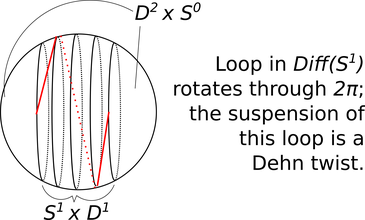# The Gromoll filtration

[2014-07-14 Mon]

In my latest preprint with Georgios Dimitroglou Rizell, we use the topology of diffeomorphism groups of high-dimensional spheres to produce interesting examples of nontrivial topology in symplectomorphism groups of cotangent bundles. Until we started thinking about this, I didn’t know much about the higher homotopy groups of $$Diff(S^n)$$ so here is some interesting stuff I learned while we were writing this paper.

The mapping class group $$\pi_0(Diff(S^n))$$ is a finite abelian group and it comes with a natural filtration by subgroups called the Gromoll filtration (thanks to Oscar Randall-Williams for telling us what it was called – once something has a name it suddenly becomes easier to find out what is known about it). Let me write this (in probably non-standard notation) as: $\cdots \subset G_k(n)\subset G_{k-1}(n)\subset\cdots\subset G_1(n)\subset G_0(n)=\pi_0(Diff(S^n))$ The subgroup $$G_k(n+k)$$ is the image of $$\pi_k(Diff(S^{n+k}))$$ under a natural homomorphism. The homomorphism is defined as follows. Think of $$S^{n+k}$$ as $$(S^n\times D^k)\cup_{S^n\times S^{k-1}} (D^n\times S^{k-1})$$. Represent an element of $$\pi_k(Diff(S^n))$$ as a map $$F\colon D^k\to Diff(S^n)$$ ($$F(y)=\phi_y\colon S^n\rightarrow S^n$$) for which $$S^{k-1}=\partial D^k$$ is sent to $$1\in Diff(S^n)$$. Now consider the map $$\Phi: S^{n+k}\to S^{n+k}$$ which equals the identity on $$D^n\times S^{k-1}$$ and equals $$\Phi(x,y)=(\phi_y(x),y))$$ on $$S^n\times D^k$$. For suitably smooth $$F$$, $$\Phi$$ is a diffeomorphism of $$S^{n+k}$$ and the map $$[F]\mapsto [\Phi]$$ is the homomorphism whose image is the subgroup $$G_k(n+k)$$ in the Gromoll filtration.

We can think of this homomorphism $$\pi_k(Diff(S^n))\rightarrow \pi_0(Diff(S^{n+k}))$$ as a “suspension” map. To understand visually what this suspension looks like, here (see Figure 1) is a familiar example in the case $$k=1$$, $$n=1$$. There is an obvious loop in $$Diff(S^1)$$ (namely the loop of rotations: $$F(y)$$ is the rotation by $$2\pi y$$ radians).Figure 1. How elements of $$G_1$$ arise from loops in diffeomorphism groups.

Suspending this loop by the above prescription gives us the Dehn twist on $$S^2$$. Unfortunately the Dehn twist on $$S^2$$ is trivial in the mapping class group, but this should give you a sense of how the diffeomorphisms in $$G_1(n)$$ arise.

In fact, $$G_1(n)$$ is completely understood. Cerf’s pseudoisotopy theorem implies that $$G_1(n)=\pi_0(Diff(S^n))$$: every mapping class of an $$n$$-sphere arises by suspending a loop of diffeomorphisms of $$S^{n-1}$$, that is “cutting along a $$S^{n-1}$$ and regluing with a twist”. But to get a nontrivial mapping class, the twist is necessarily more complicated than the loop of rotations.

Nontrivial elements in higher homotopy groups are sometimes fiddly to get one's hands on; suspending an element $$\alpha\in\pi_k(Diff(S^{n}))$$ and looking at its image in the mapping class group of an $$(n+k)$$-sphere, then using that mapping class as a gluing map to stick two discs together and make an exotic $$(n+k+1)$$-sphere lets you turn $$\alpha$$ into a manifold ($$S^{n+k+1}_{\alpha}$$ in our notation), from which it is maybe easier to extract an invariant to prove nontriviality. For example, if the resulting exotic sphere does not bound a parallelisable manifold then the original homotopy class must have been nontrivial. This (via an incredible theorem of Abouzaid) is the way the Gromoll filtration enters into our work: if a homotopy class survives in $$G_k(n+k)$$ modulo the subgroup of $$G_k(n+k)$$ consisting of exotic spheres bounding parallelisable manifolds then we can use the homotopy class to construct nontrivial topology in the symplectomorphism group of $$T^*S^n$$.

So it is important for us to know when $$G_k(n+k)$$ is nontrivial. Fortunately, there are many interesting calculations of the higher groups $$G_k$$ in the literature, providing a convenient way to detect nontrivial higher homotopy in the diffeomorphism groups of spheres.

Comments, corrections and contributions are very welcome; please drop me an email at j.d.evans at lancaster.ac.uk if you have something to share.

CC-BY-SA 4.0 Jonny Evans.